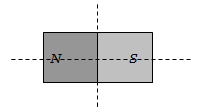Two bar magnets with magnetic moments 2 M and M are fastened together at right angles to each other at their centres to form a crossed system, which can rotate freely about a vertical axis through the centre. The crossed system sets in earth’s magnetic field with magnet having magnetic moment 2M making an angle $\theta$ with the magnetic meridian such that

(a) $\theta ={\mathrm{tan}}^{-1}\left(\frac{1}{\sqrt{3}}\right)$            (b) $\theta ={\mathrm{tan}}^{-1}\left(\sqrt{3}\right)$

(c) $\theta ={\mathrm{tan}}^{-1}\left(\frac{1}{2}\right)$               (d) $\theta ={\mathrm{tan}}^{-1}\left(\frac{3}{4}\right)$

Concept Questions :-

Earth's magnetism
High Yielding Test Series + Question Bank - NEET 2020

Difficulty Level:

The angle of dip at a certain place is 30o. If the horizontal component of the earth’s magnetic field is H, the intensity of the total magnetic field is

1. $\frac{H}{2}$                            2. $\frac{2H}{\sqrt{3}}$

3. $H\sqrt{2}$                         4. $H\sqrt{3}$

Concept Questions :-

Earth's magnetism
High Yielding Test Series + Question Bank - NEET 2020

Difficulty Level:

The time period of oscillation of a freely suspended bar magnet with usual notations is given by

1. $T=2\mathrm{\pi }\sqrt{\frac{\mathrm{I}}{{\mathrm{MB}}_{\mathrm{H}}}}$

2.  $T=2\mathrm{\pi }\sqrt{\frac{M{B}_{H}}{I}}$

3. $T=2\sqrt{\frac{\mathrm{I}}{{\mathrm{MB}}_{\mathrm{H}}}}$

4. $T=2\mathrm{\pi }\sqrt{\frac{{B}_{H}}{MI}}$

Concept Questions :-

Bar magnet
High Yielding Test Series + Question Bank - NEET 2020

Difficulty Level:

Time period for a magnet is T. If it is divided in four equal parts along its axis and perpendicular to its axis as shown then time period for each part will be1. 4T

2. T/4

3. T/2

4. T

Concept Questions :-

Bar magnet
High Yielding Test Series + Question Bank - NEET 2020

Difficulty Level:

The period of oscillation of a magnet in vibration magnetometer is 2 sec. The period of oscillation of a magnet whose magnetic moment is four times that of the first magnet is

(a) 1 sec                          (b) 4 sec

(c) 8 sec                          (d) 0.5 sec

Concept Questions :-

Earth's magnetism
High Yielding Test Series + Question Bank - NEET 2020

Difficulty Level:

A magnetic needle is made to vibrate in uniform field H, then its time period is T. If it vibrates in the field of intensity 4H, its time period will be

(a) 2T                             (b) T/2

(c) 2/T                            (d) T

Concept Questions :-

Earth's magnetism
High Yielding Test Series + Question Bank - NEET 2020

Difficulty Level:

A magnet is suspended in such a way that it oscillates in the horizontal plane. It makes 20 oscillations per minute at a place where dip angle is 30o and 15 oscillations per minute at a place where dip angle is 60o. The ratio of total earth's magnetic field at the two places is

1. $3\sqrt{3}:8$                      2. $16:9\sqrt{3}$

3. 4:9                           4. $2\sqrt{3}:9$

Concept Questions :-

Analogy between electrostatics and magnetostatics
High Yielding Test Series + Question Bank - NEET 2020

Difficulty Level:

A bar magnet A of magnetic moment MA is found to oscillate at a frequency twice that of magnet B having identical size as magnet A, of magnetic moment MB when placed in a vibrating magneto-meter. We may say that

(a) ${M}_{A}=2{M}_{B}$                  (b) MA = 8MB

(c) MA = 4MB                  (d) MB = 8MA

Concept Questions :-

Bar magnet
High Yielding Test Series + Question Bank - NEET 2020

Difficulty Level:

A magnet of magnetic moment M oscillating freely in earth's horizontal magnetic field makes n oscillations per minute. If the magnetic moment is quadrupled and the earth's field is doubled, the number of oscillations made per minute would be

1. $\frac{n}{2\sqrt{2}}$                         2. $\frac{n}{\sqrt{2}}$

3. $2\sqrt{2}n$                        4. $\sqrt{2}n$

Concept Questions :-

Bar magnet
High Yielding Test Series + Question Bank - NEET 2020

Difficulty Level:

Magnets A and B are geometrically similar but the magnetic moment of A is twice that of B. If T1 and T2 be the time periods of the oscillation when their like poles and unlike poles are kept together respectively, then $\frac{{T}_{1}}{{T}_{2}}$ will be

(a) 1/3                              (b) 1/2

(c) $1}{\sqrt{3}}$                          (d) $\sqrt{3}$

Concept Questions :-

Bar magnet